Скачать презентацию Rigidity of 2 d and 3 d Pinned

d40a5a1345f7cace525c67c81dd95972.ppt

• Количество слайдов: 26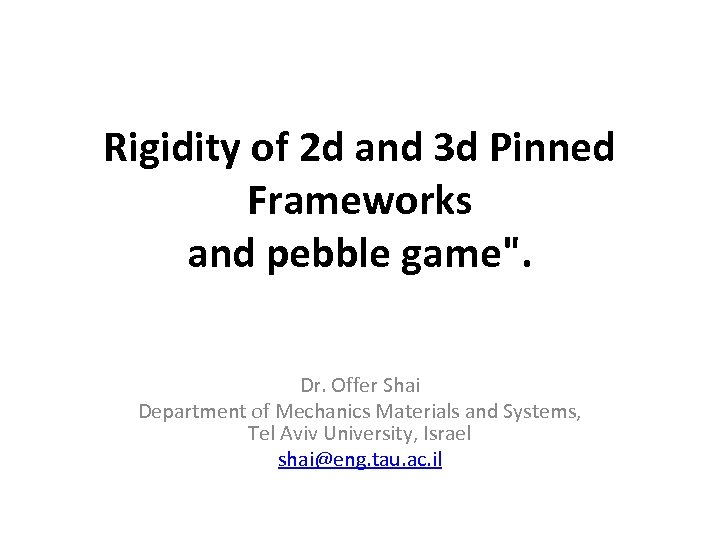Rigidity of 2 d and 3 d Pinned Frameworks and pebble game". Dr. Offer Shai Department of Mechanics Materials and Systems, Tel Aviv University, Israel [email protected] tau. ac. il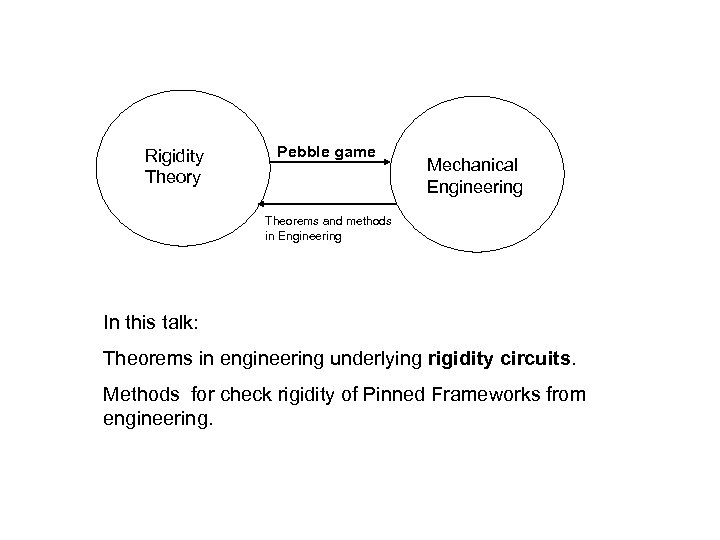Rigidity Theory Pebble game Mechanical Engineering Theorems and methods in Engineering In this talk: Theorems in engineering underlying rigidity circuits. Methods for check rigidity of Pinned Frameworks from engineering.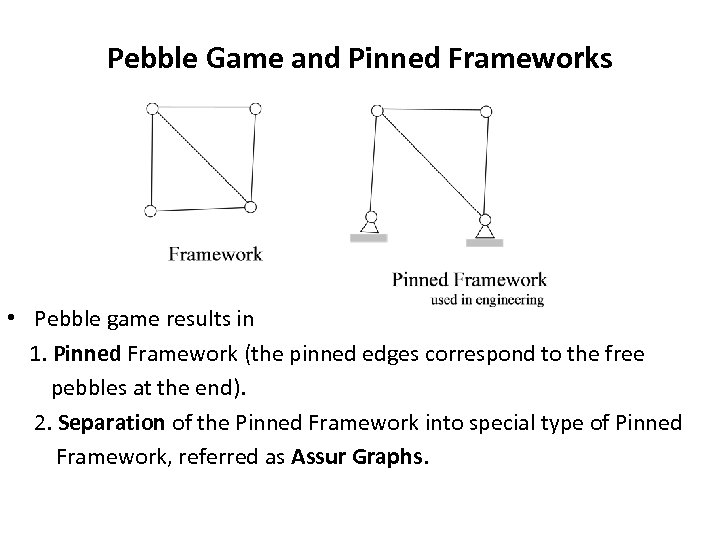Pebble Game and Pinned Frameworks • Pebble game results in 1. Pinned Framework (the pinned edges correspond to the free pebbles at the end). 2. Separation of the Pinned Framework into special type of Pinned Framework, referred as Assur Graphs.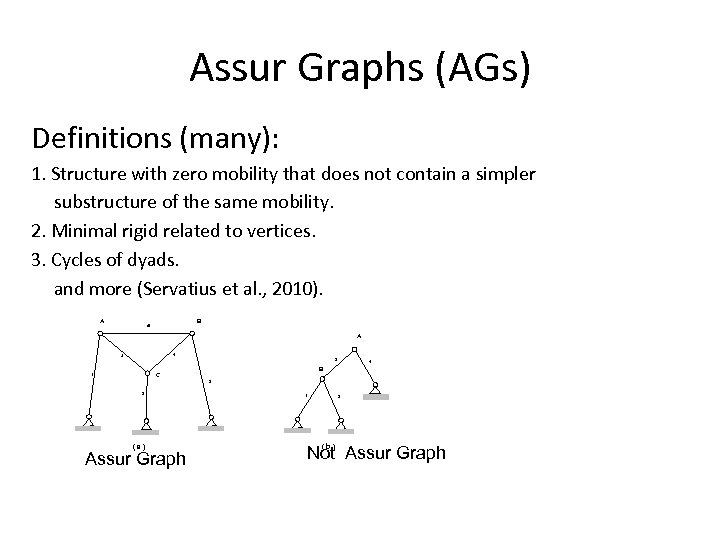Assur Graphs (AGs) Definitions (many): 1. Structure with zero mobility that does not contain a simpler substructure of the same mobility. 2. Minimal rigid related to vertices. 3. Cycles of dyads. and more (Servatius et al. , 2010). A B 6 A 4 5 3 4 B C 1 3 2 (a) Assur Graph 1 2 (b ) Not Assur Graph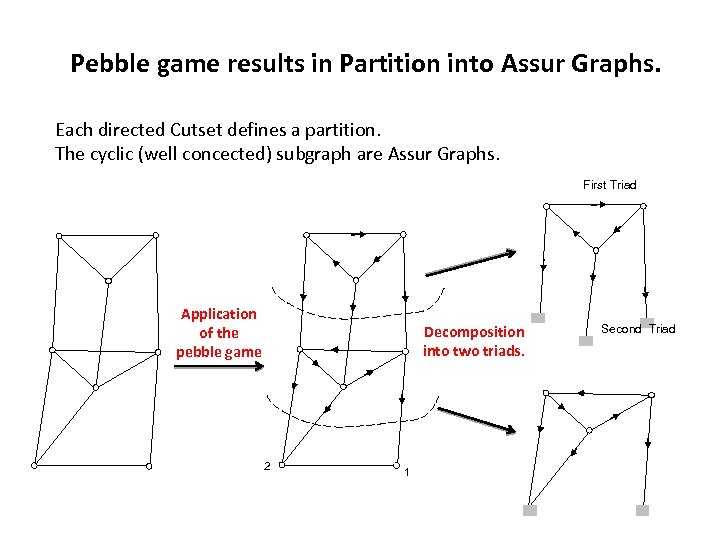Pebble game results in Partition into Assur Graphs. Each directed Cutset defines a partition. The cyclic (well concected) subgraph are Assur Graphs. First Triad Application of the pebble game Decomposition into two triads. 2 1 Second Triad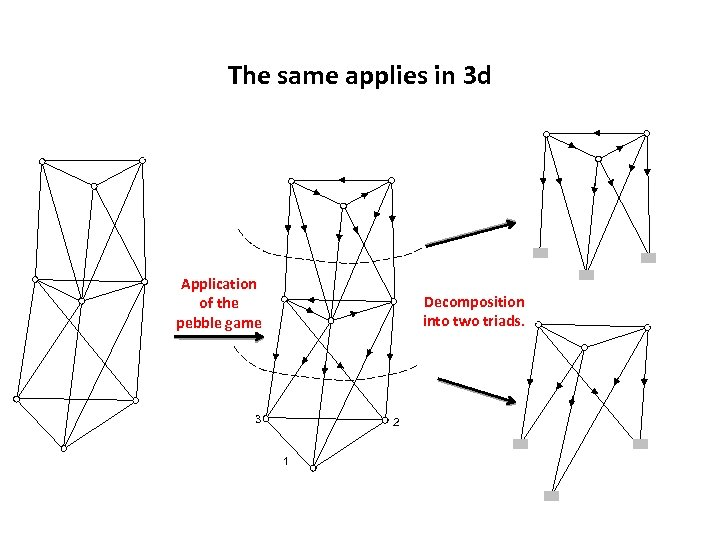The same applies in 3 d Application of the pebble game Decomposition into two triads. 3 2 1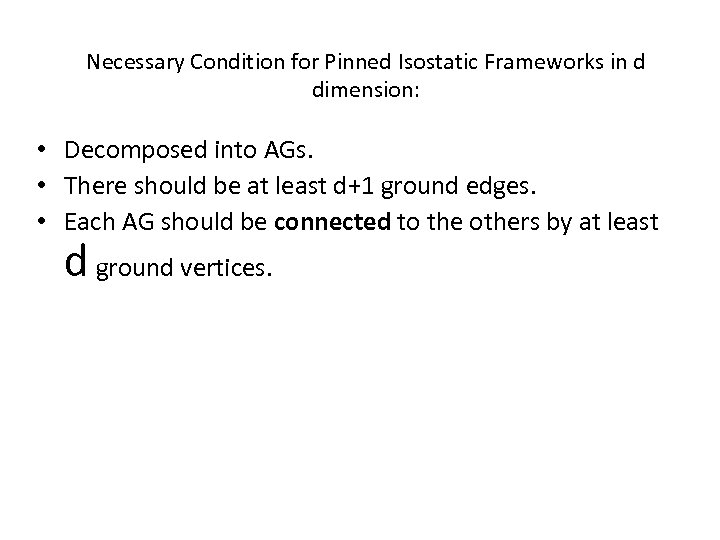Necessary Condition for Pinned Isostatic Frameworks in d dimension: • Decomposed into AGs. • There should be at least d+1 ground edges. • Each AG should be connected to the others by at least d ground vertices.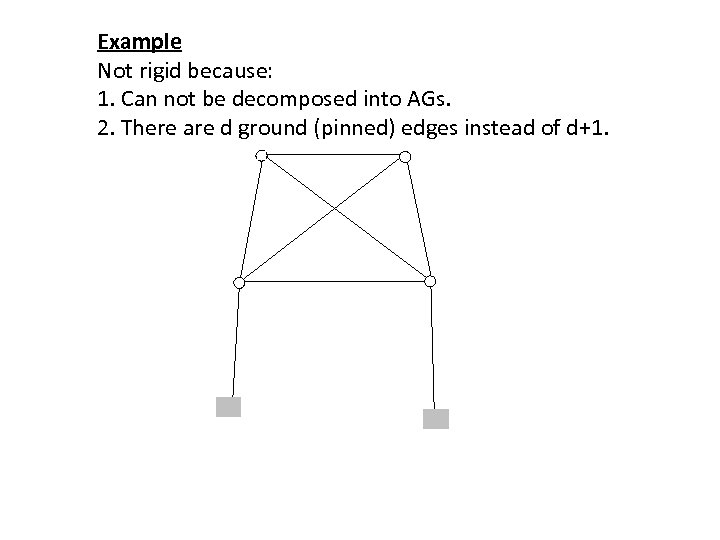Example Not rigid because: 1. Can not be decomposed into AGs. 2. There are d ground (pinned) edges instead of d+1.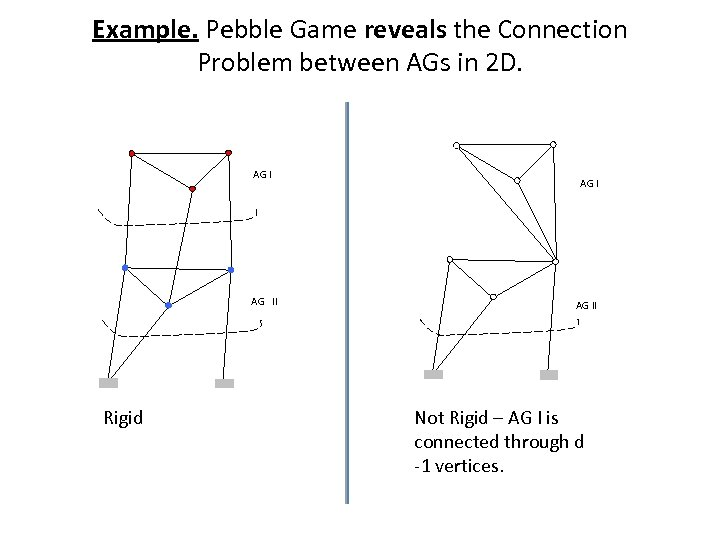Example. Pebble Game reveals the Connection Problem between AGs in 2 D. AG I AG II Rigid AG II Not Rigid – AG I is connected through d -1 vertices.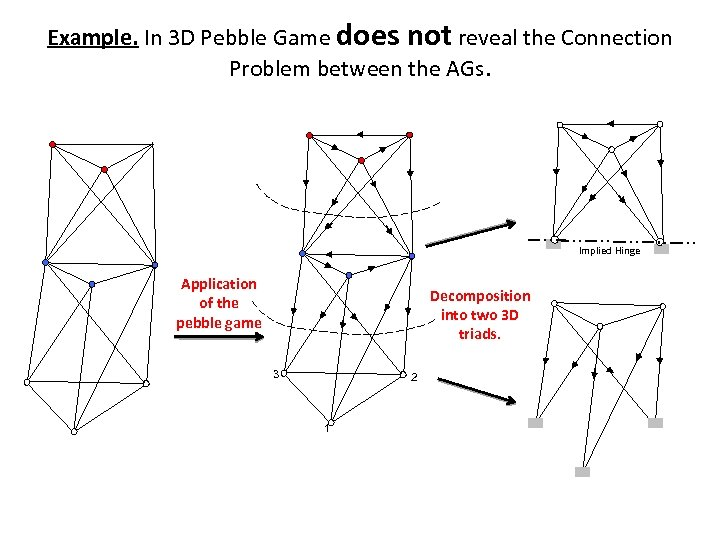Example. In 3 D Pebble Game does not reveal the Connection Problem between the AGs. Implied Hinge Application of the pebble game Decomposition into two 3 D triads. 3 2 1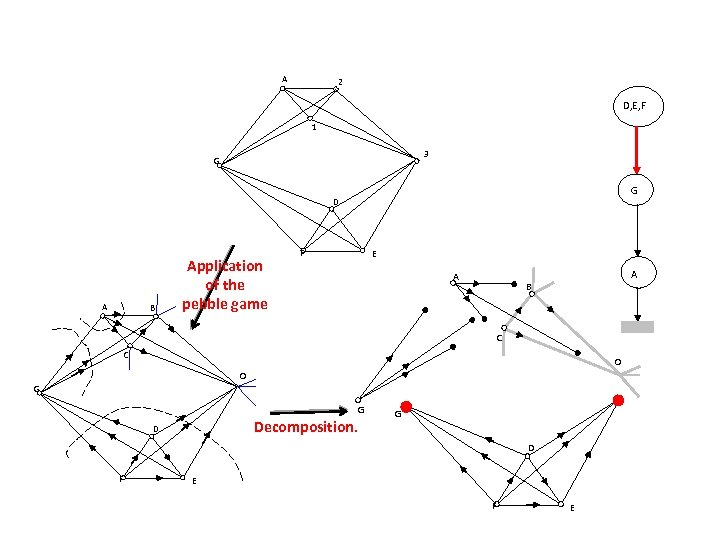A 2 D, E, F 1 3 G G D A B Application of the pebble game F E A A B C C O O G G Decomposition. D G D F E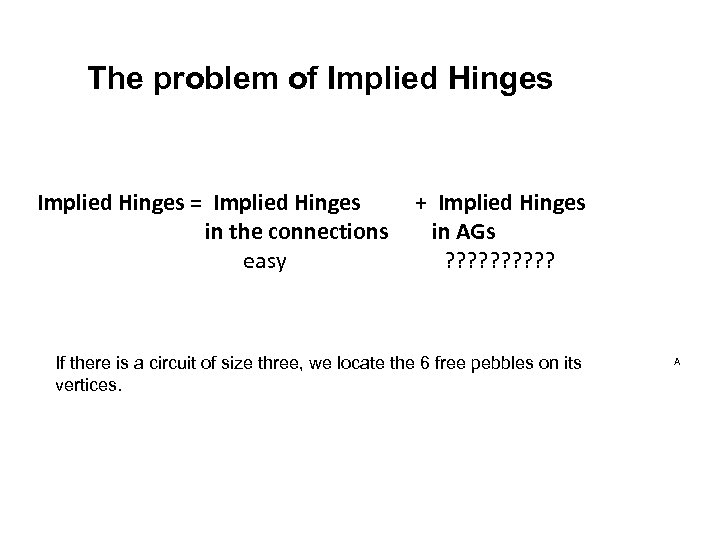The problem of Implied Hinges = Implied Hinges + Implied Hinges in the connections in AGs easy ? ? ? ? ? If there is a circuit of size three, we locate the 6 free pebbles on its vertices. A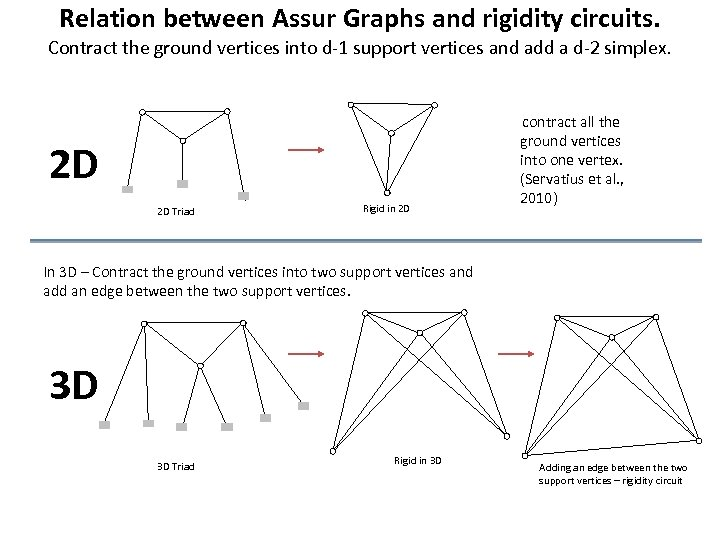Relation between Assur Graphs and rigidity circuits. Contract the ground vertices into d-1 support vertices and add a d-2 simplex. 2 D 2 D Triad Rigid in 2 D contract all the ground vertices into one vertex. (Servatius et al. , 2010) In 3 D – Contract the ground vertices into two support vertices and add an edge between the two support vertices. 3 D 3 D Triad Rigid in 3 D Adding an edge between the two support vertices – rigidity circuit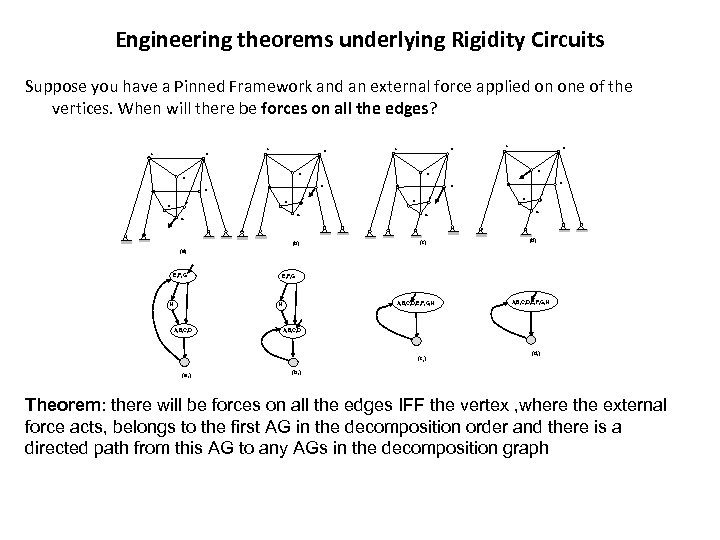Engineering theorems underlying Rigidity Circuits Suppose you have a Pinned Framework and an external force applied on one of the vertices. When will there be forces on all the edges? A A B A B B H H C D C D F E F E G G G (b) (c) (d) (a) E, F, G H E, F, G A, B, C, D, E, F, G, H H A, B, C, D (c 1) (a 1) A, B, C, D, E, F, G, H (d 1) (b 1) Theorem: there will be forces on all the edges IFF the vertex , where the external force acts, belongs to the first AG in the decomposition order and there is a directed path from this AG to any AGs in the decomposition graph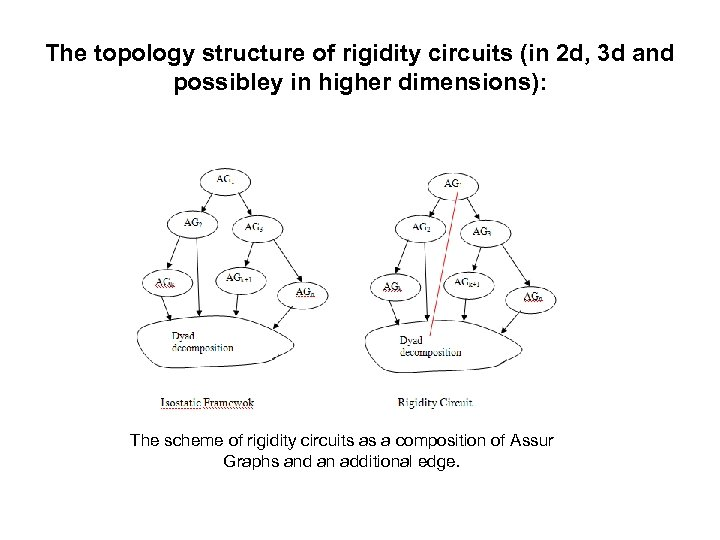The topology structure of rigidity circuits (in 2 d, 3 d and possibley in higher dimensions): The scheme of rigidity circuits as a composition of Assur Graphs and an additional edge.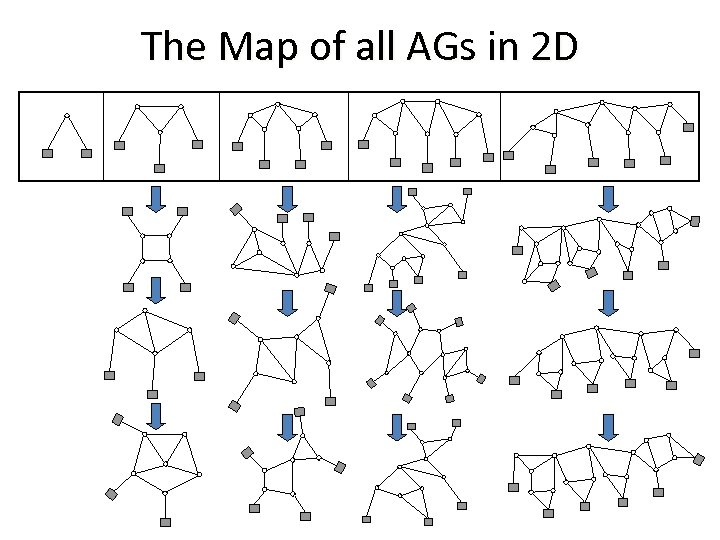The Map of all AGs in 2 D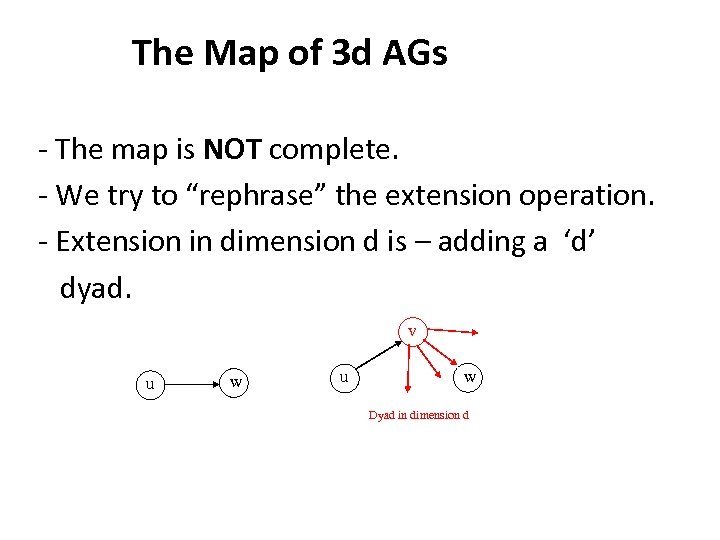The Map of 3 d AGs - The map is NOT complete. - We try to “rephrase” the extension operation. - Extension in dimension d is – adding a ‘d’ dyad. v u w Dyad in dimension d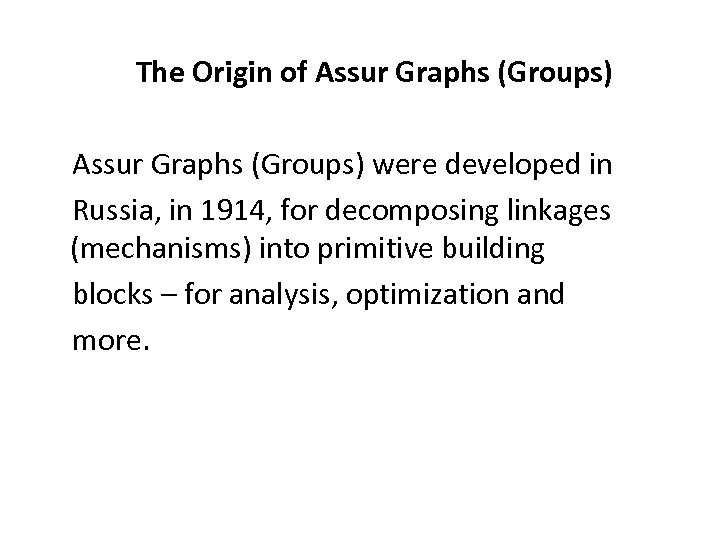The Origin of Assur Graphs (Groups) were developed in Russia, in 1914, for decomposing linkages (mechanisms) into primitive building blocks – for analysis, optimization and more.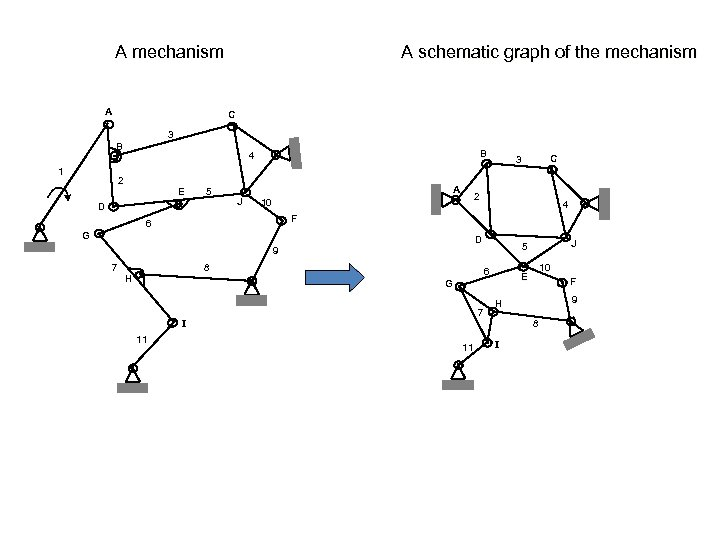A mechanism A A schematic graph of the mechanism C 3 B 1 B 4 2 E 5 D A J 10 2 4 F 6 G D 9 7 8 H 6 7 I 11 J 5 10 E G 11 C 3 F 9 H 8 I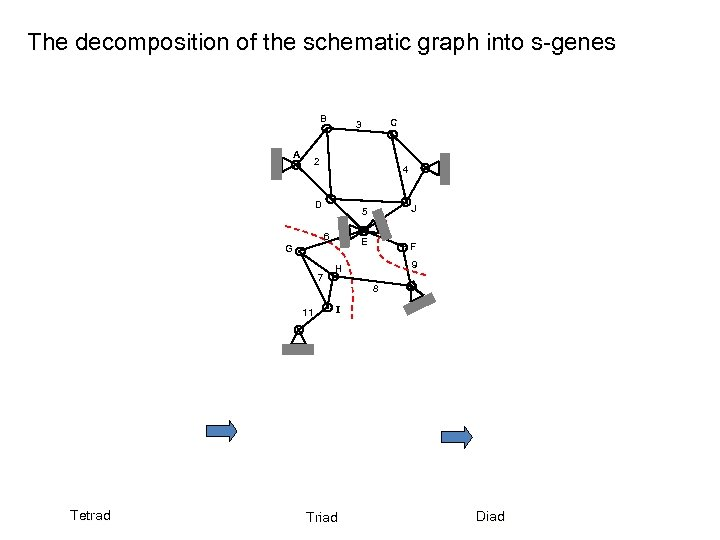The decomposition of the schematic graph into s-genes B A 2 4 D 6 7 11 J 5 10 E G Tetrad C 3 F 9 H 8 I Triad Diad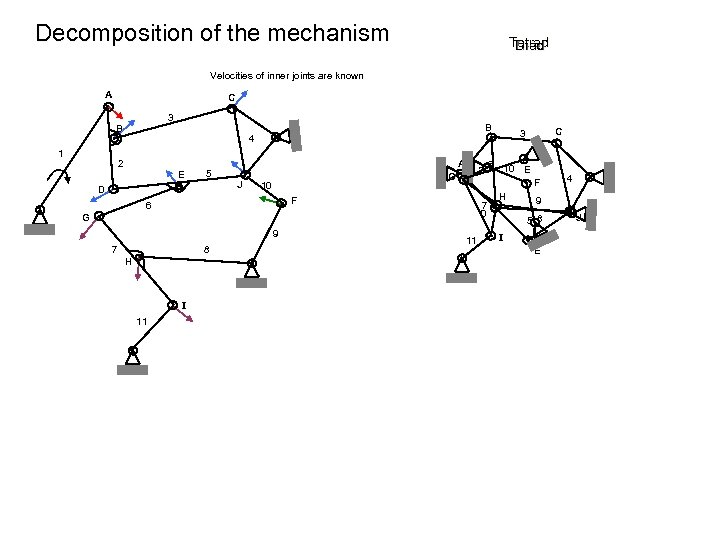Decomposition of the mechanism Tetrad Diad Triad Velocities of inner joints are known A C 3 B 1 B 4 2 E 5 D A J 2 G 10 7 D G 9 7 8 H I 11 6 10 E F F 6 C 3 11 H 4 9 5 8 I E J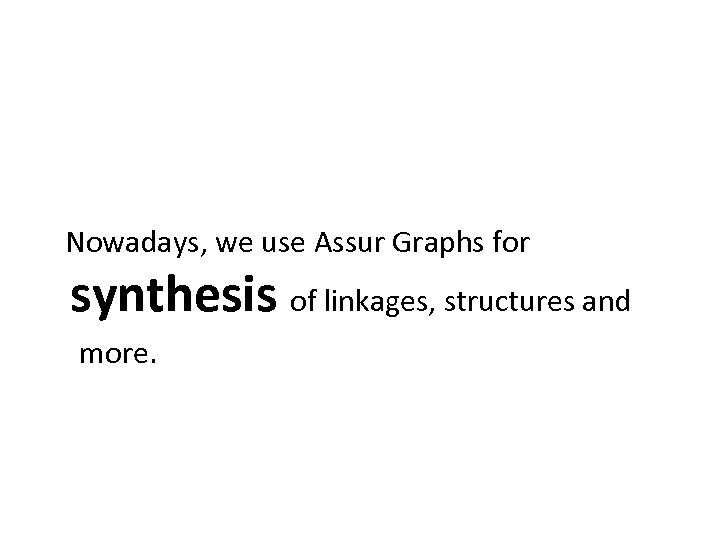Nowadays, we use Assur Graphs for synthesis of linkages, structures and more.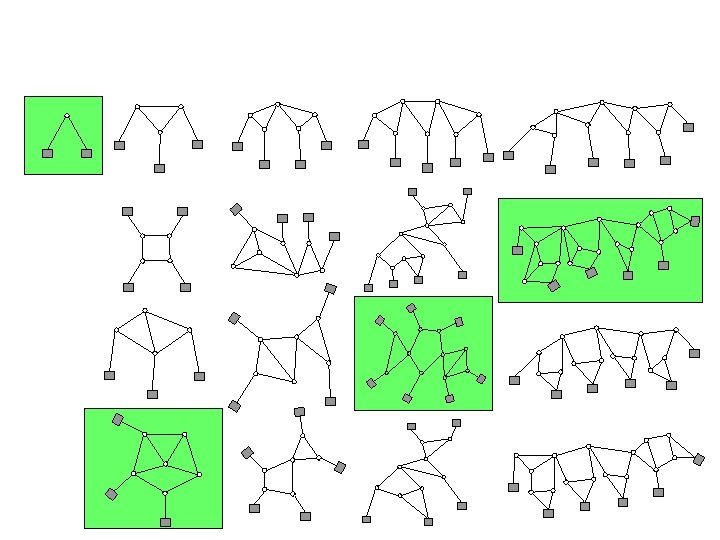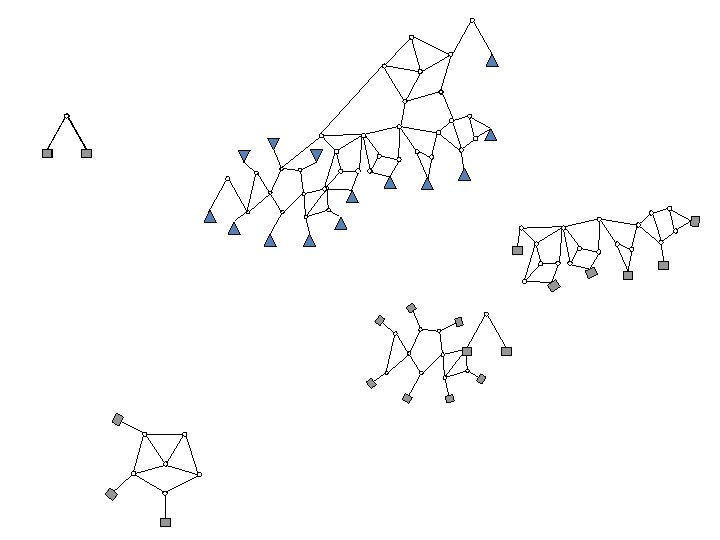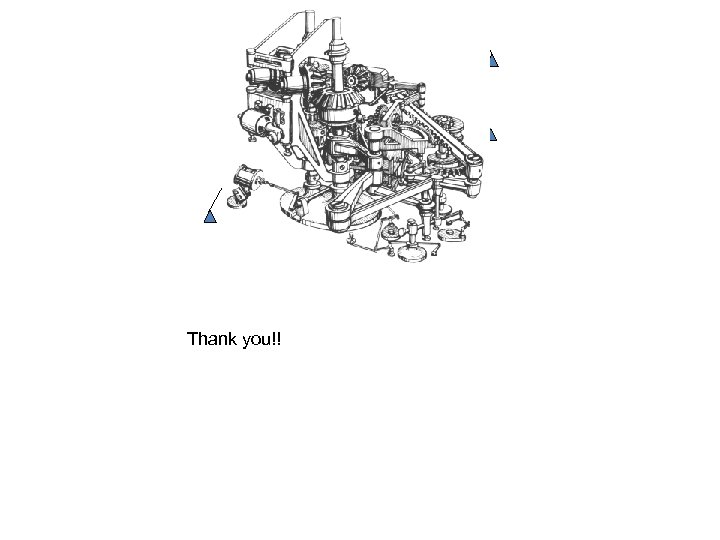Thank you!!